# Some pandas notes (part 1, EDA)

Teaching my son python at the moment – when I gave him a final project to do on his own, he complained and said he did not remember how to do one step from the prior lessons.

In the end, I am guessing I really only have maybe 30 commands in any language memorized, and then maybe another 70 I know of (but often need to look at the docs for its less often used arguments).

Here I figured it would be good for learners for me to put down my notes on pandas dataframes in python. At least the less than 20 commands I regularly use.

So first of I think a 3 part series, is using pandas for exploratory data analysis (EDA). Besides these tables I will show here, I often only use histograms and smooth conditional plots. Those I don’t even use very often.

Debated on writing a post that EDA is overrated, (I spend way more time understanding business objectives, then tuning a reasonably non-linear machine learning model and seeing its results dominates after that point). So it makes writing a post on EDA quite easy though – I can show a dozen pandas methods I regularly use and that is over 90% of the typical EDA work I do.

Here I will show an example using the PPP loan data available to download, in particular I will be using the over 150k loan data. A nice aspect of pandas is you can read in a CSV file directly from a url.

``````# Python code examples for EDA
import pandas as pd

# PPP loans, see full dataset
# at https://data.sba.gov/dataset/ppp-foia

# Can read in from url directly
pp_loans.shape # over 900k rows``````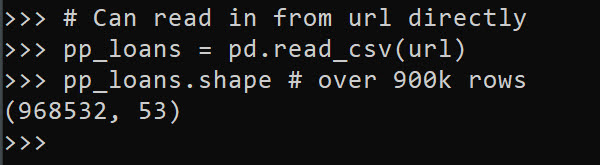At work I am often querying a database using SQLAlchemy instead of reading a csv file. But this is a nice example to show off – the data is too big to really work effectively in Excel, but pandas it should be no problem for most peoples machines.

So you can see the first thing I look at is `data.shape` to get the number of rows and columns. Next I often just print the columns using `list(data)`:

``````# Can use list to see all of the variables
list(pp_loans)``````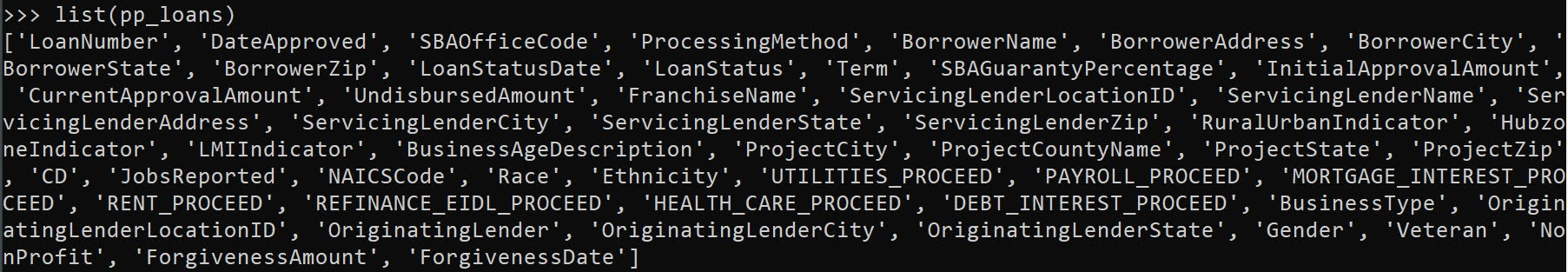So you can access/modify the actual column names via `data.columns`, but this is a bit nicer printing.

Next I often summarize the data using the `data.describe()` method. I like transposing this result to be easier to read though.

``````# Transpose describe for easier reading
pp_loans.describe().T``````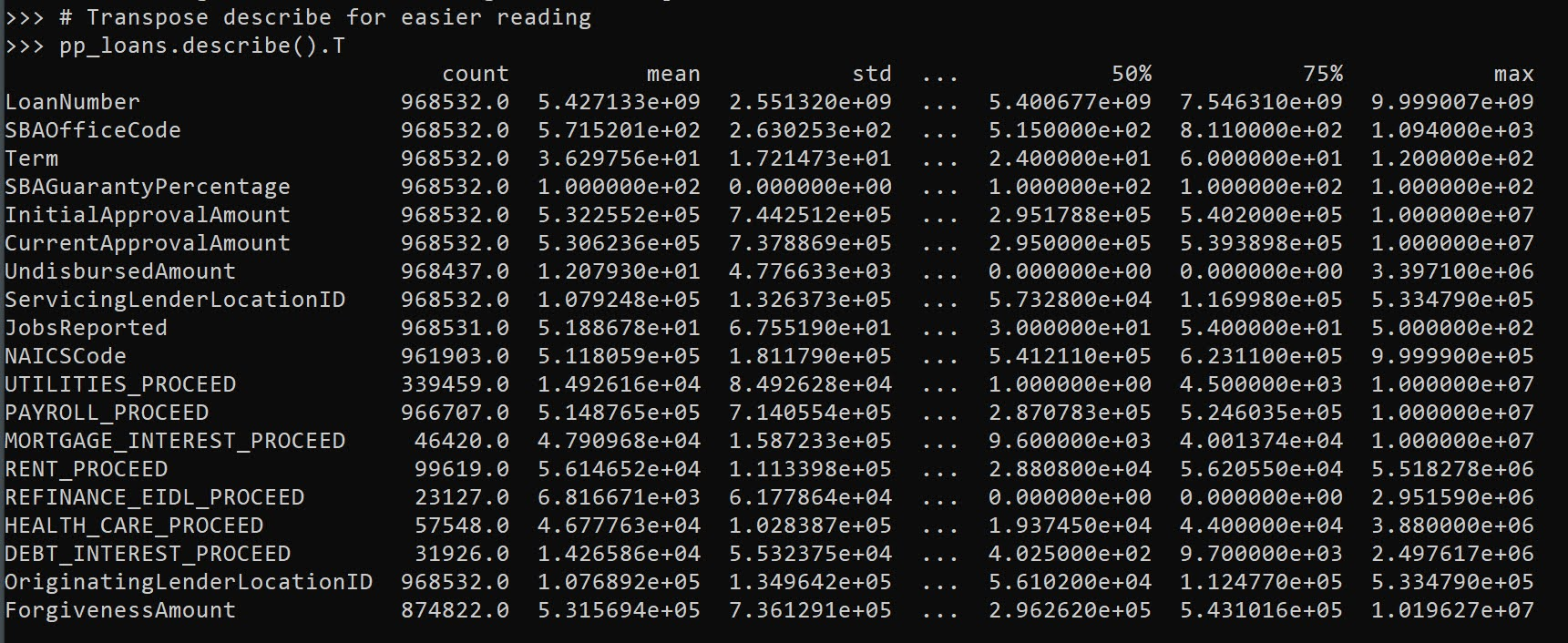Here we get a bit of a mess of scientific notation (also this only shows summaries of float data by default, not categorical or dates). If you want to set a bit easier on the eyes printing, I typically have this `pd.set_option()` handy. Also you can scale the data, here I divide everything by 1000.

``````# If you dont want scientific notation
pd.set_option('display.float_format', '{:,.0f}'.format)
pp_loans.describe().T/1000``````

One of the things I like about this the most is the count, so you can see data that has missing values (that here should likely be set to 0 for the `*_PROCEED` variables). The second thing I look at are the min/max. Sometimes you can spot rogue missing values or censoring in the data. Then looking at the quartiles is typically informative as to how skewed the data is. Only then do I bother with the mean – which for binary data is reasonably informative.

You can see for this particular data, it is the loans over \$150k – here this means apparently the `CurrentApprovalAmount`, as 150k to 10million (the apparent cap on the PPP loans). So this is \$150k conditional on approval dataset.

The next step I am typically looking the distribution of various categorical data. For that I am often just using `data['variable'].value_counts()`:

# Value counts is maybe my favorite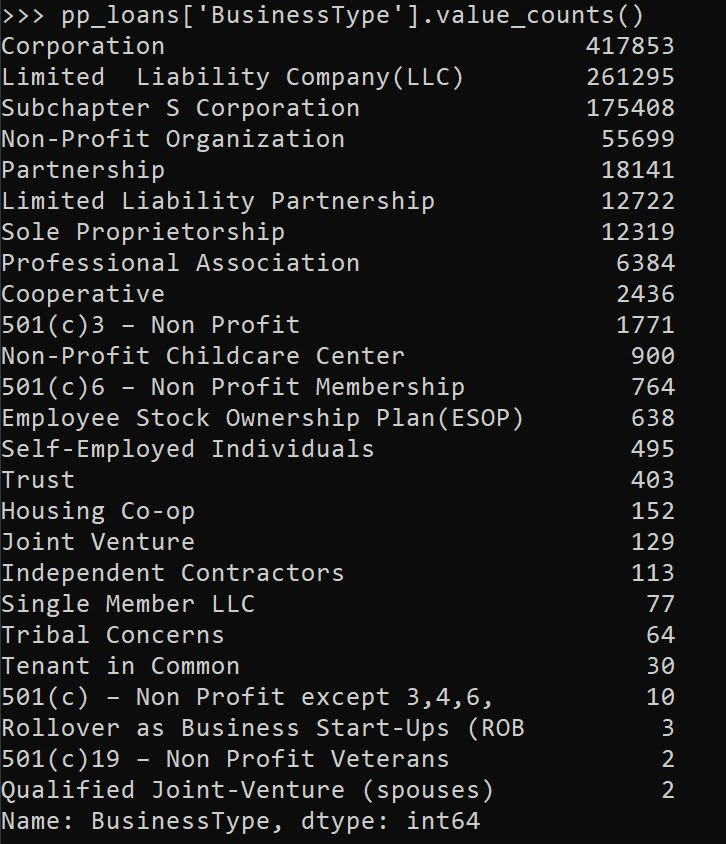This prints out all of the business types in this example, but with a very large number will limit to the head/tail. Here we can see Corps are the most common, with LLCs and s-corps as the next.

The next most common pandas method I use is `data.groupby()`, this produces summary aggregations conditional on different groups in the data. For example, here we can do the total sum of the loans (per million dollars) for each of the business types.

``````# Groupby with stats is next, per million
y = 'CurrentApprovalAmount'
pp_loans.groupby(x)[y].sum().sort_values()/1e6``````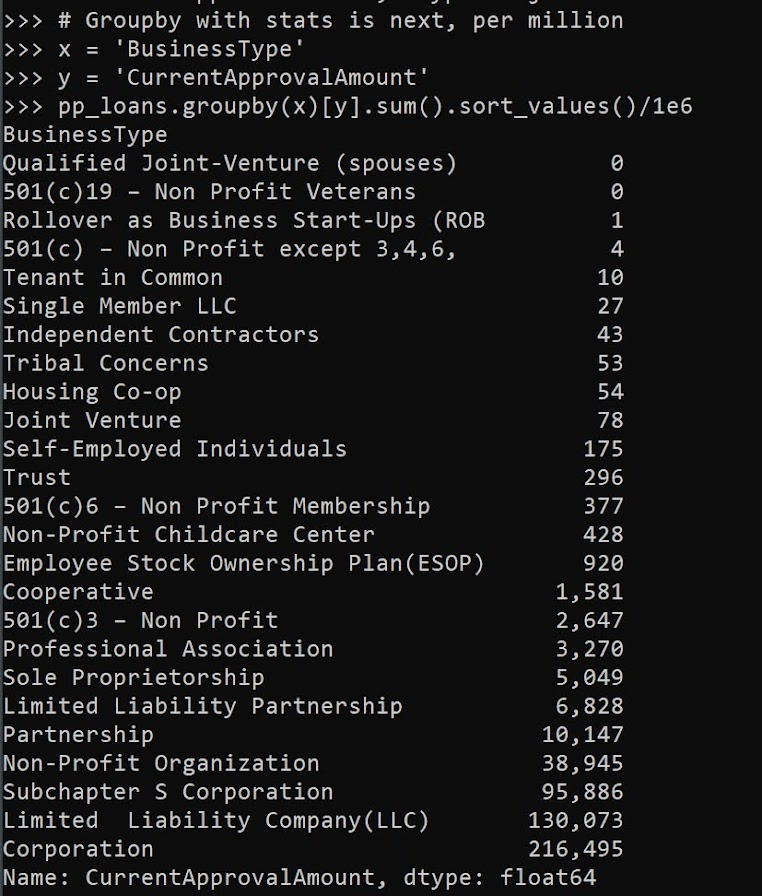You can see that you can chain multiple pandas methods together, which is just a typical python object oriented pattern. So I tack on a `.sort_values()` to the end of the groupby. Note again these are in millions of dollars, so Coop’s got over a billion dollars of PPP loans in this data (it will be more overall, these again are only loans over \$150k, the link at the beginning has a bunch of additional datasets with smaller loan amounts).

You can subsequently do additional functions in groupby, for just an example here I create my own normal distribution confidence interval of the mean for large samples.

``````# Can do more complicated functions
def ci95(x):
mx = x.mean()
sx = x.std()
res = pd.DataFrame([[mx - 2*sx,mx + 2*sx]])
res.columns = ['low','high']
return res

pp_loans.groupby(x)[y].apply(ci95)``````

I often use this for functions with binary data, but otherwise we can again chain methods together, so we can use `data.groupby(x)[y].describe()` to get those summaries for each of our groups. Also as an FYI you can pass in multiple `y` values to summarize via a list, although it gets a bit hairy in the output:

``````# And can have multiple outcome variables
ys = [y,'PAYROLL_PROCEED']
pp_loans.groupby(x)[ys].describe()``````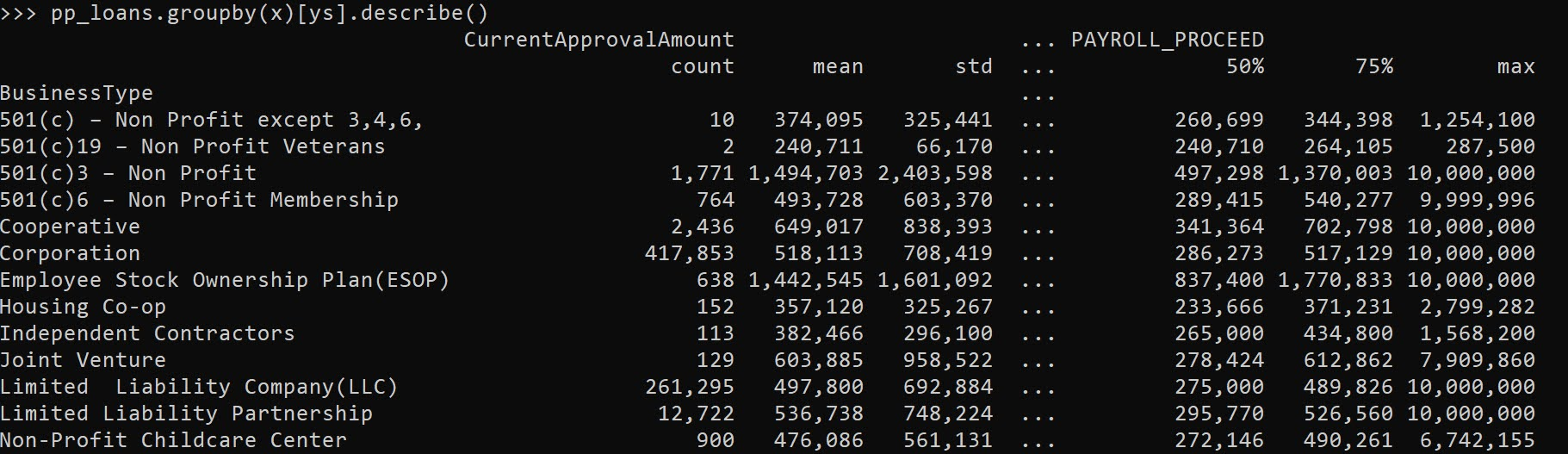One of the common things I do at this point is to simply dump the results to a CSV file I can lookat, e.g. something like:

``````# Can chain together and save result to csv
pp_loans.groupby(x)[ys].describe().to_csv('aggstats.csv')``````

Or assign to a new object, and some python editors (such as Spyder) have a data browsers.

The final EDA part I use most often is simply sorting the dataset and looking at the head/tail.

``````# Can sort values and look at head/tail
pp_loans.sort_values(by=ys,ascending=False,inplace=True,ignore_index=True)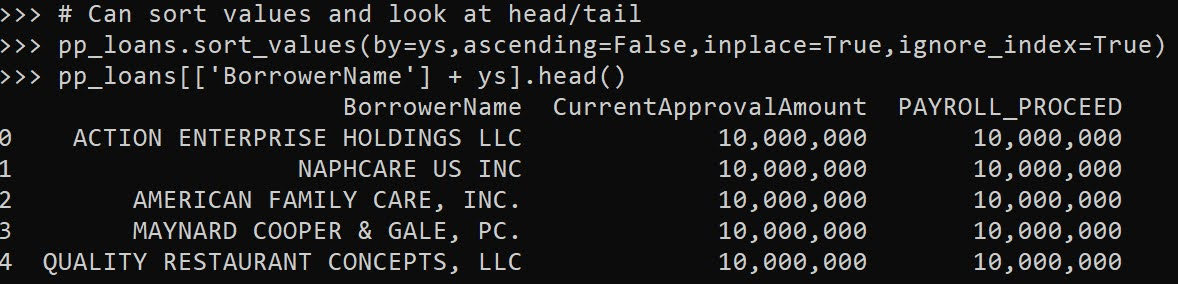I often will export the head of the data, e.g. something like `data.head().to_csv('checkdata.csv')` to view all of the fields and their content as well when first figuring out fields, how often they are filled in, etc.